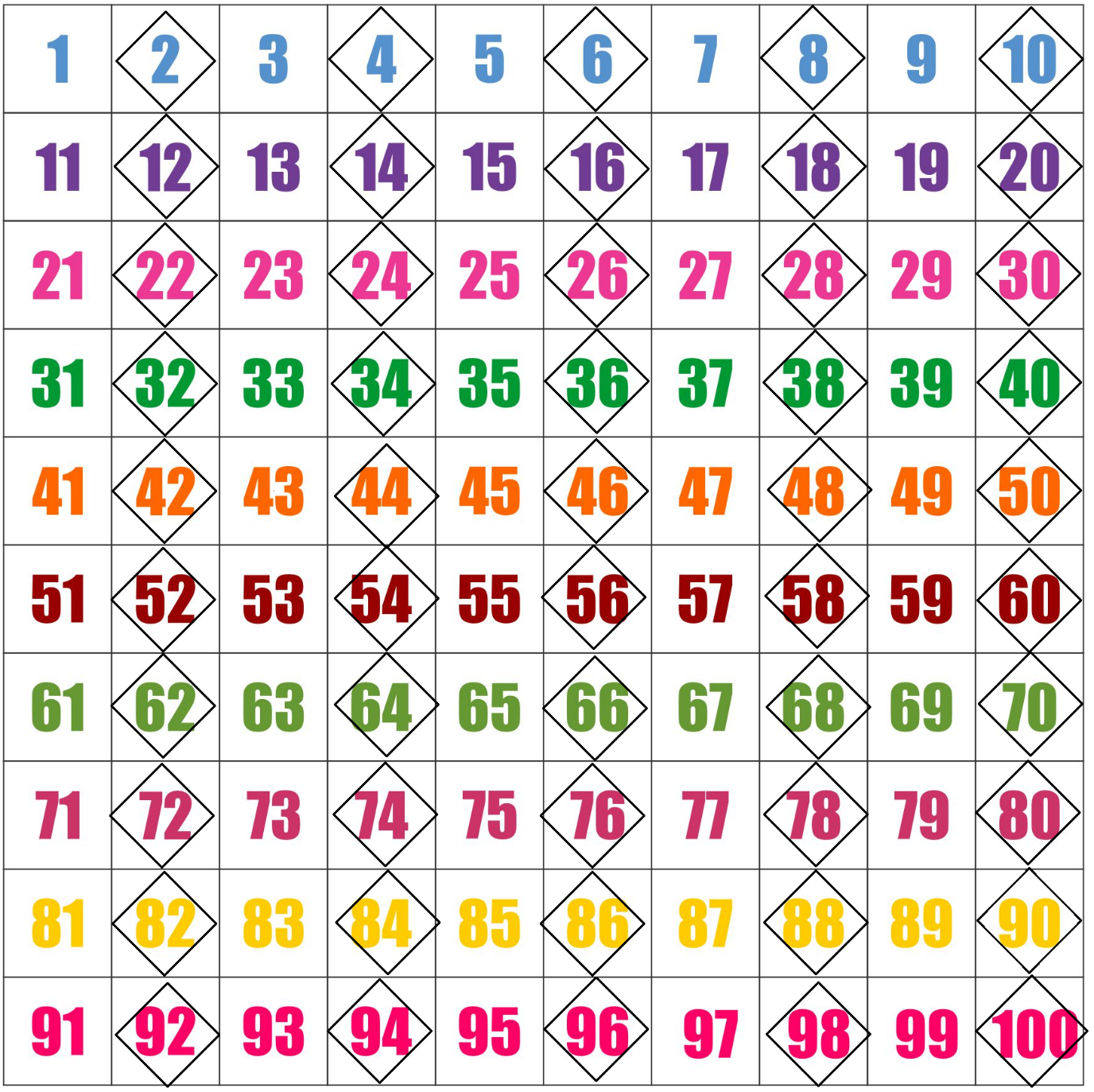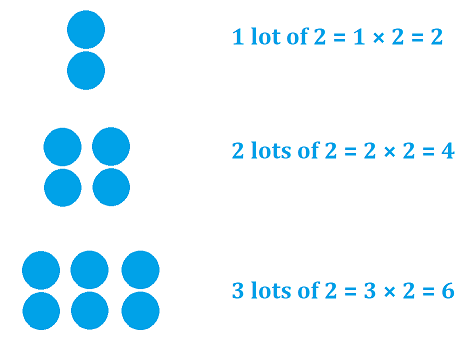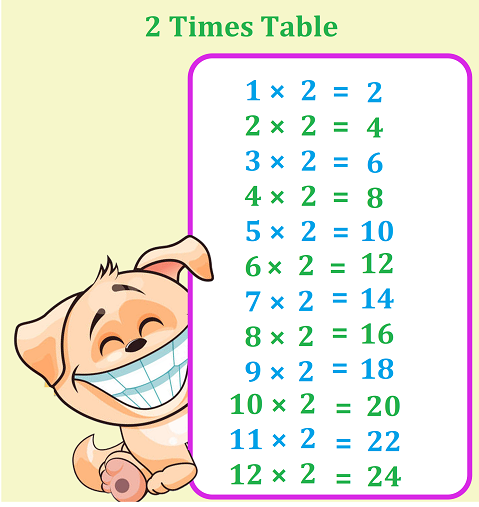# Math > Year 2

## 2 time’s table

There are different ways of completing 2 times table.

Counting in 2s
To work 2 times table, we can count in 2s starting from 0.

Here is a counting pattern:
2, 4, 6, 8, 10, 12, 14, 16, 18 …

This pattern goes up in twos.All the numbers in 2 times table are even numbers.
Even numbers are the numbers that can be divided by 2.

Groups of 22 Times tableExample: Olive bought 2 packs of candles. In each pack, there are 6 candles. How many candles did she buy?

Number of candles in 1 pack = 2
Number of packs bought by Olive = 6
Total number of candles = 6 × 2 = 12

//